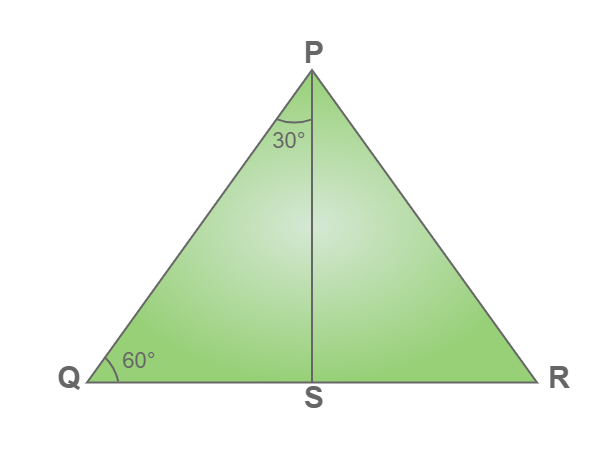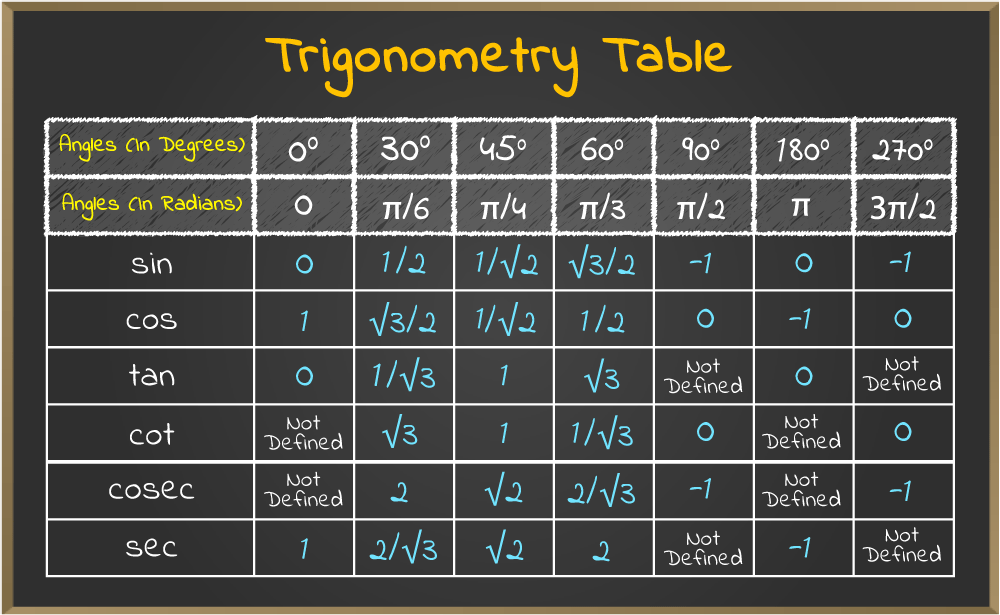GFG App
Open AppBrowser
Continue

The value of tan 30 degrees is 1/2. In radian tan 30° is written as tan π/6. Trigonometric functions are very important, for various studies such as it is useful to study Wave motion, Movement of light, the study velocity of harmonic oscillators, and other applications. In fraction form, the value of tan 30° is 1/√3, and in decimal form, the value is 0.577

tan 30° = 1/√3

## What is the Value of Tan 30 degrees?

The value of tan 30 degrees in decimals is 0.577. Converting degree to radian, that is, θ (radians) = θ × π/180° The value of tan 30 degrees is calculated by various methods, including the formula of the trigonometric ratio as we know that tan x = Perpendicular / Base. In a right-angled triangle, ABC with angle A be 30°

Tan A = Tan 30° = 1/√3

## Tan 30 Degrees in Terms of Trigonometric Functions

Trigonometric functions are also called circular functions or trigonometric ratios. The relationship between angles and sides is represented by these trigonometric functions. The representation of the value of tan 30° using trigonometric functions are:

• Tan 30° = ± √(sec2 30° – 1)
• Tan 30° = 1/cot 30°

## Derivation of Tan 30 Degree (Geometrical Method)

Consider an equilateral triangle PQR where PQ = QR = RP and ∠P = ∠Q = ∠R = 60°. …………….(1)Draw a perpendicular bisector PS of the line QR from vertex P.

Now, QS = RS, and PS = √3QS

In right angle triangle PQS

tan 30° = Perpendicular / Base

here,
Perpendicular = QS
Base = PS

tan 30° = QS / PS

tan 30° = 1/√3

Thus, the value of tan 30° is 1/√3

## Value of Trigonometric Functions

Various values of trigonometric functions are used to solve complex functions. Basic values of trigonometric functions can be learned from the tale below:## Solved Examples on Tan 30 Degree

Example 1: In a right-angle triangle, one angle is 30°, and the base for 30° is 3m. Find the length of the perpendicular.

Solution:

Given:  Base = 3m

Tan 30 = 1/√3

P/B = 1/√3

P/3 = 1/√3

p = √3

Example 2: In a right triangle hypotenuse is 20 cm, and one side is 10√3 cm, find the angles of the triangle.

Solution:

Given: H = 20, and B = 10√3

Finding third side using pythagoras theorem.

P2 + B2 = H

P2 + (10√3)2 = 202

p2 + 300 = 400

P2 = 100

p = 10

The third side is 10cm. The ratio of the two sides 10cm and 10√3cm is 1/√3,  So there must be an angle of 30° in triangle Since the triangle is a right angle, so the third angle is

90° – 30° = 60°

The Angles of a triangle are 30°, 60°, 90°.

## FAQs on Tan 30 Degree

Question 1: Write the value of tan 30°.

The value of tan 30 degrees is 1/√3

Question 2: Write the value of 30 degrees in radians.

The value of 30 degrees in radian is π/6.

Question 3: Write the value of sin 30° and cos 30°.

The value of sin 30° is 1/2 and the value of cos 30° is √3/2

Question 4: Write the formula for the tan function.

In a right-angle triangle tan of an angle, is equal to the ratio of the opposite side of the angle and the base of a right-angle triangle.

i.e tan A = Opposite Side(Perpendicular)/Base Side.

Question 5: How to find the value of tan 30 degrees from a practical approach?